# Chapter 4 Greedy Algorithms Slides by Kevin Wayne

• Slides: 24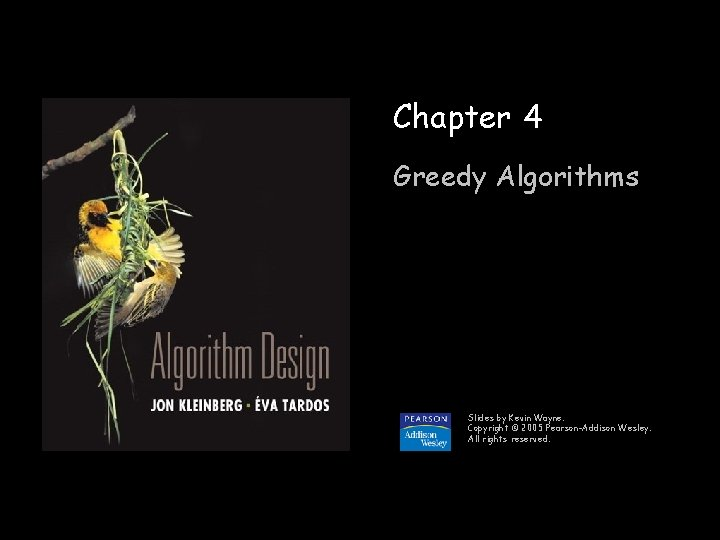4. 5 Minimum Spanning TreeMinimum Spanning Tree Minimum spanning tree. Given a connected graph G = (V, E) with realvalued edge weights ce, an MST is a subset of the edges T E such that T is a spanning tree whose sum of edge weights is minimized. 24 4 23 6 16 4 18 5 9 5 11 8 14 10 9 6 7 8 11 7 21 G = (V, E) T, e T ce = 50 Cayley's Theorem. There are nn-2 spanning trees of Kn. can't solve by brute force 3Applications MST is fundamental problem with diverse applications. n n Network design. – telephone, electrical, hydraulic, TV cable, computer, road Approximation algorithms for NP-hard problems. – traveling salesperson problem, Steiner tree Indirect applications. – max bottleneck paths – LDPC codes for error correction – image registration with Renyi entropy – learning salient features for real-time face verification – reducing data storage in sequencing amino acids in a protein – model locality of particle interactions in turbulent fluid flows – autoconfig protocol for Ethernet bridging to avoid cycles in a network Cluster analysis. 4Greedy Algorithms Kruskal's algorithm. Start with T = . Consider edges in ascending order of cost. Insert edge e in T unless doing so would create a cycle. Reverse-Delete algorithm. Start with T = E. Consider edges in descending order of cost. Delete edge e from T unless doing so would disconnect T. Prim's algorithm. Start with some root node s and greedily grow a tree T from s outward. At each step, add the cheapest edge e to T that has exactly one endpoint in T. Remark. All three algorithms produce an MST. 5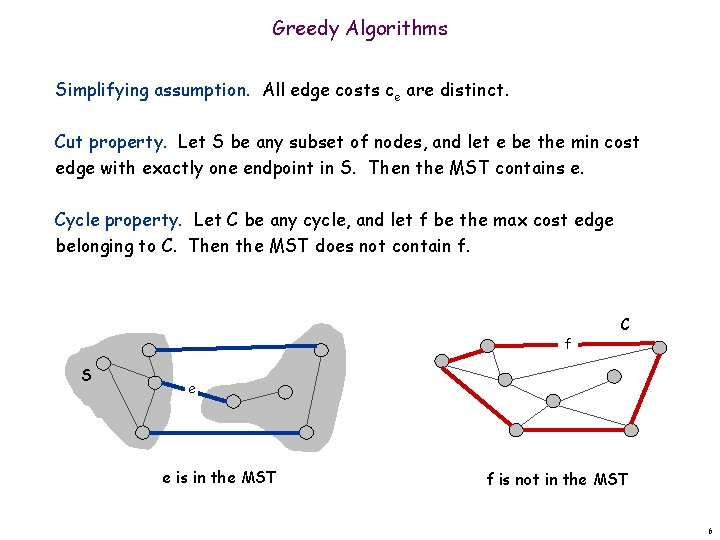Greedy Algorithms Simplifying assumption. All edge costs ce are distinct. Cut property. Let S be any subset of nodes, and let e be the min cost edge with exactly one endpoint in S. Then the MST contains e. Cycle property. Let C be any cycle, and let f be the max cost edge belonging to C. Then the MST does not contain f. f S C e e is in the MST f is not in the MST 6Cycles and Cuts Cycle. Set of edges the form a-b, b-c, c-d, …, y-z, z-a. 1 2 3 6 4 Cycle C = 1 -2, 2 -3, 3 -4, 4 -5, 5 -6, 6 -1 5 8 7 Cutset. A cut is a subset of nodes S. The corresponding cutset D is the subset of edges with exactly one endpoint in S. 1 2 3 6 Cut S = { 4, 5, 8 } Cutset D = 5 -6, 5 -7, 3 -4, 3 -5, 7 -8 4 5 7 8 7Cycle-Cut Intersection Claim. A cycle and a cutset intersect in an even number of edges. 2 1 3 6 Cycle C = 1 -2, 2 -3, 3 -4, 4 -5, 5 -6, 6 -1 Cutset D = 3 -4, 3 -5, 5 -6, 5 -7, 7 -8 Intersection = 3 -4, 5 -6 4 5 8 7 Pf. (by picture) C S V-S 8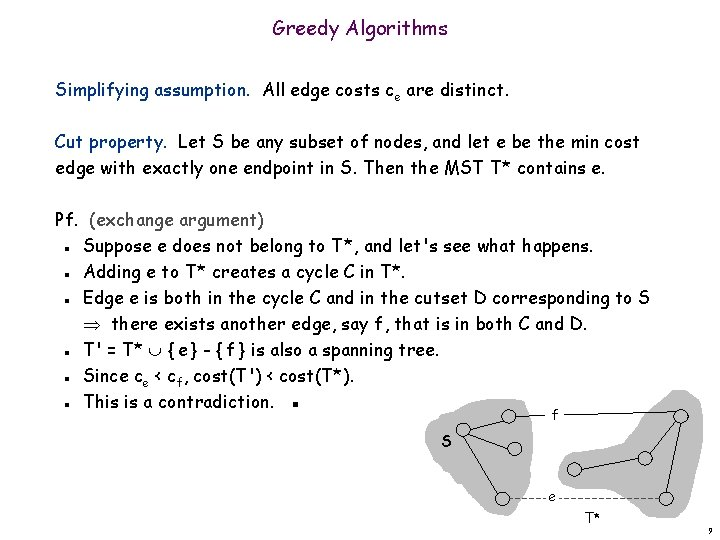Greedy Algorithms Simplifying assumption. All edge costs ce are distinct. Cut property. Let S be any subset of nodes, and let e be the min cost edge with exactly one endpoint in S. Then the MST T* contains e. Pf. (exchange argument) Suppose e does not belong to T*, and let's see what happens. Adding e to T* creates a cycle C in T*. Edge e is both in the cycle C and in the cutset D corresponding to S there exists another edge, say f, that is in both C and D. T' = T* { e } - { f } is also a spanning tree. Since ce < cf, cost(T') < cost(T*). This is a contradiction. ▪ n n n f S e T* 9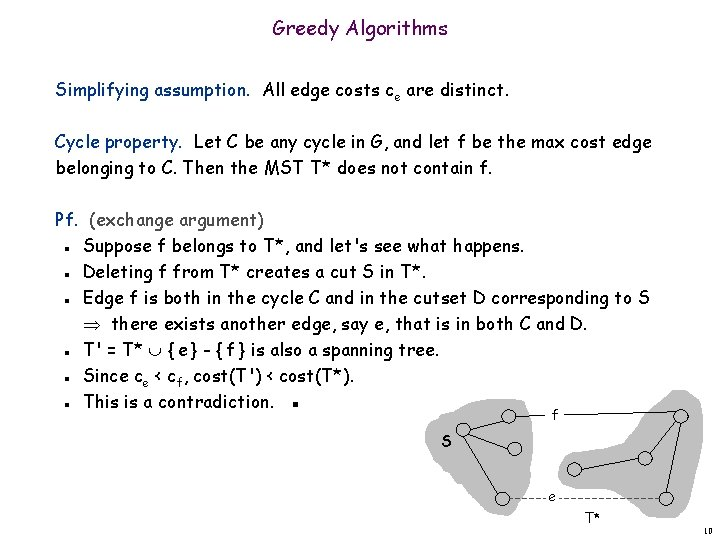Greedy Algorithms Simplifying assumption. All edge costs ce are distinct. Cycle property. Let C be any cycle in G, and let f be the max cost edge belonging to C. Then the MST T* does not contain f. Pf. (exchange argument) Suppose f belongs to T*, and let's see what happens. Deleting f from T* creates a cut S in T*. Edge f is both in the cycle C and in the cutset D corresponding to S there exists another edge, say e, that is in both C and D. T' = T* { e } - { f } is also a spanning tree. Since ce < cf, cost(T') < cost(T*). This is a contradiction. ▪ n n n f S e T* 10Prim's Algorithm: Proof of Correctness Prim's algorithm. [Jarník 1930, Dijkstra 1957, Prim 1959] Initialize S = any node. Apply cut property to S. Add min cost edge in cutset corresponding to S to T, and add one new explored node u to S. n n n S 11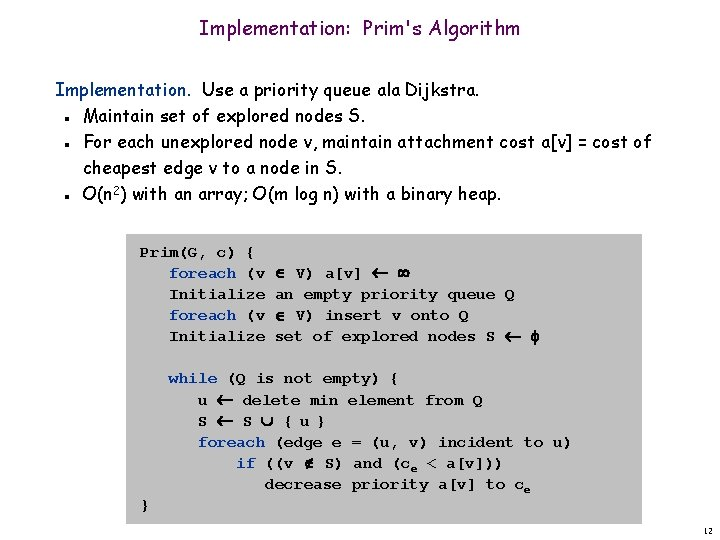Implementation: Prim's Algorithm Implementation. Use a priority queue ala Dijkstra. Maintain set of explored nodes S. For each unexplored node v, maintain attachment cost a[v] = cost of cheapest edge v to a node in S. O(n 2) with an array; O(m log n) with a binary heap. n n n Prim(G, c) { foreach (v Initialize V) a[v] an empty priority queue Q V) insert v onto Q set of explored nodes S while (Q is not empty) { u delete min element from Q S S {u} foreach (edge e = (u, v) incident to u) if ((v S) and (ce < a[v])) decrease priority a[v] to ce } 12Kruskal's Algorithm: Proof of Correctness Kruskal's algorithm. [Kruskal, 1956] Consider edges in ascending order of weight. Case 1: If adding e to T creates a cycle, discard e according to cycle property. Case 2: Otherwise, insert e = (u, v) into T according to cut property where S = set of nodes in u's connected component. n n n v e Case 1 S e u Case 2 13Implementation: Kruskal's Algorithm Implementation. Use the union-find data structure. Build set T of edges in the MST. Maintain set for each connected component. O(m log n) for sorting and O(m (m, n)) for union-find. n n n m n 2 log m is O(log n) essentially a constant Kruskal(G, c) { Sort edges weights so that c 1 c 2 . . . cm. T foreach (u V) make a set containing singleton u are u and v in different connected components? for i = 1 to m (u, v) = ei if (u and v are in different sets) { T T {ei} merge the sets containing u and v } merge two components return T } 14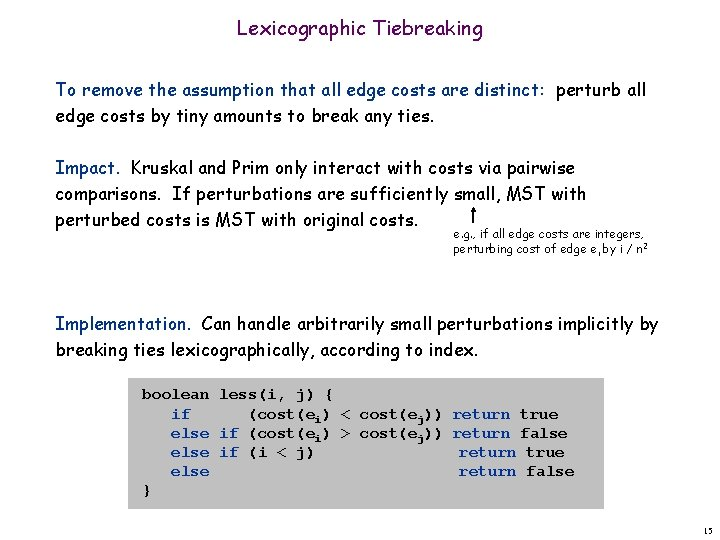Lexicographic Tiebreaking To remove the assumption that all edge costs are distinct: perturb all edge costs by tiny amounts to break any ties. Impact. Kruskal and Prim only interact with costs via pairwise comparisons. If perturbations are sufficiently small, MST with perturbed costs is MST with original costs. e. g. , if all edge costs are integers, perturbing cost of edge ei by i / n 2 Implementation. Can handle arbitrarily small perturbations implicitly by breaking ties lexicographically, according to index. boolean less(i, j) { if (cost(ei) < cost(ej)) return true else if (cost(ei) > cost(ej)) return false else if (i < j) return true else return false } 15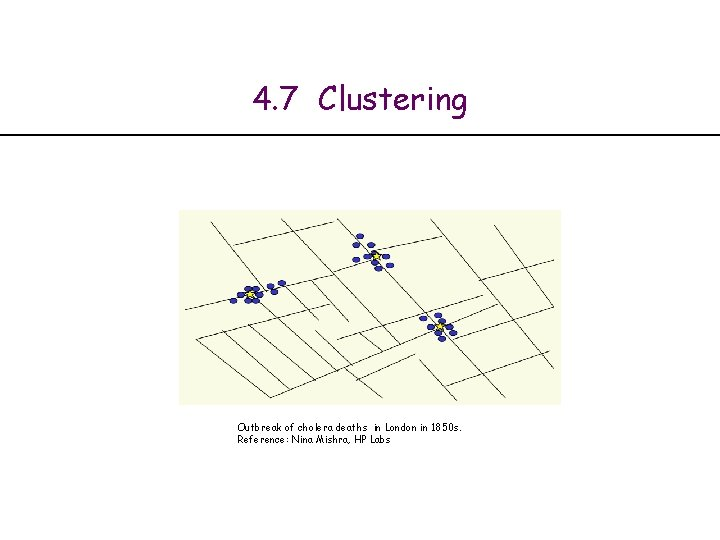4. 7 Clustering Outbreak of cholera deaths in London in 1850 s. Reference: Nina Mishra, HP LabsClustering. Given a set U of n objects labeled p 1, …, pn, classify into coherent groups. photos, documents. micro-organisms Distance function. Numeric value specifying "closeness" of two objects. number of corresponding pixels whose intensities differ by some threshold Fundamental problem. Divide into clusters so that points in different clusters are far apart. Routing in mobile ad hoc networks. Identify patterns in gene expression. Document categorization for web search. Similarity searching in medical image databases Skycat: cluster 109 sky objects into stars, quasars, galaxies. n n n 17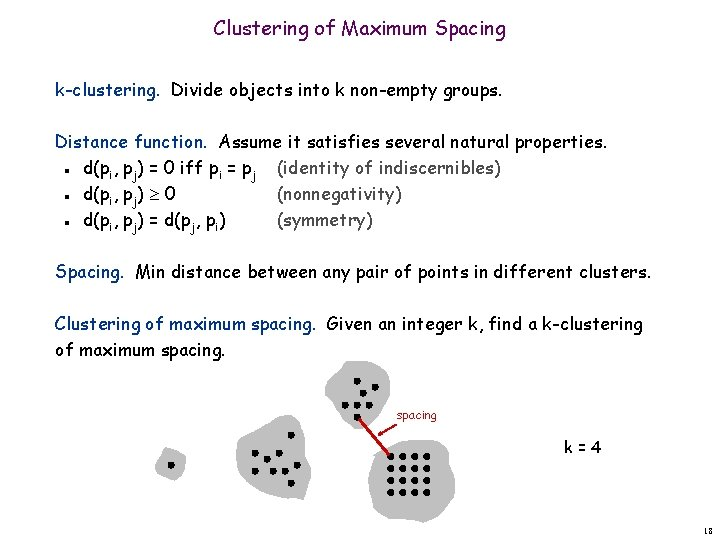Clustering of Maximum Spacing k-clustering. Divide objects into k non-empty groups. Distance function. Assume it satisfies several natural properties. d(pi, pj) = 0 iff pi = pj (identity of indiscernibles) d(pi, pj) 0 (nonnegativity) d(pi, pj) = d(pj, pi) (symmetry) n n n Spacing. Min distance between any pair of points in different clusters. Clustering of maximum spacing. Given an integer k, find a k-clustering of maximum spacing k=4 18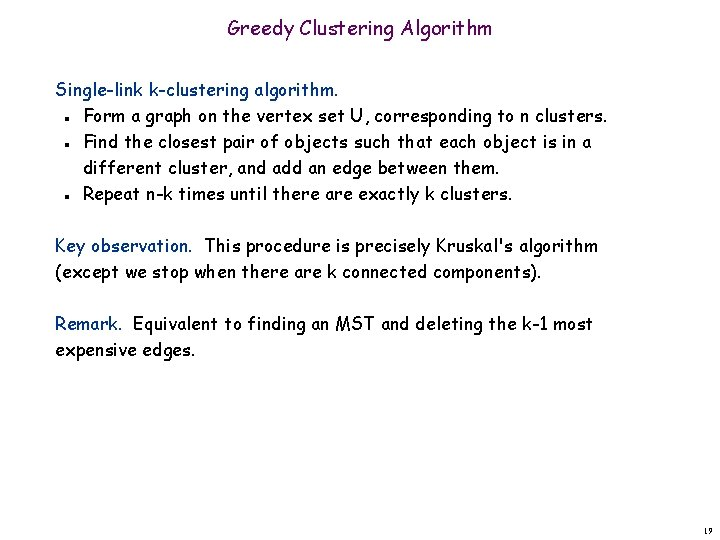Greedy Clustering Algorithm Single-link k-clustering algorithm. Form a graph on the vertex set U, corresponding to n clusters. Find the closest pair of objects such that each object is in a different cluster, and add an edge between them. Repeat n-k times until there are exactly k clusters. n n n Key observation. This procedure is precisely Kruskal's algorithm (except we stop when there are k connected components). Remark. Equivalent to finding an MST and deleting the k-1 most expensive edges. 19Greedy Clustering Algorithm: Analysis Theorem. Let C* denote the clustering C*1, …, C*k formed by deleting the k-1 most expensive edges of a MST. C* is a k-clustering of max spacing. Pf. Let C denote some other clustering C 1, …, Ck. The spacing of C* is the length d* of the (k-1)st most expensive edge. Let pi, pj be in the same cluster in C*, say C*r, but different clusters in C, say Cs and Ct. Some edge (p, q) on pi-pj path in C*r spans two different clusters in C. All edges on pi-pj path have length d* since Kruskal chose them. Ct Cs Spacing of C is d* since p and q are in different clusters. ▪ C* n n n r pi p q pj 20Extra Slides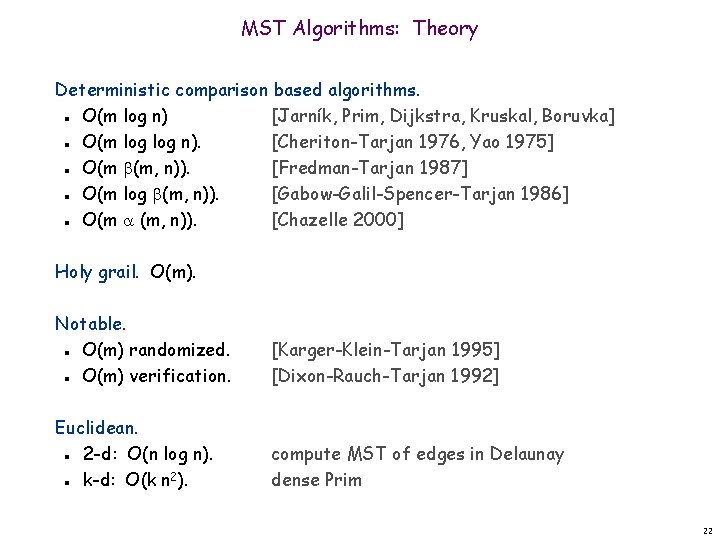MST Algorithms: Theory Deterministic comparison based algorithms. O(m log n) [Jarník, Prim, Dijkstra, Kruskal, Boruvka] O(m log n). [Cheriton-Tarjan 1976, Yao 1975] O(m (m, n)). [Fredman-Tarjan 1987] O(m log (m, n)). [Gabow-Galil-Spencer-Tarjan 1986] O(m (m, n)). [Chazelle 2000] n n n Holy grail. O(m). Notable. O(m) randomized. O(m) verification. [Karger-Klein-Tarjan 1995] [Dixon-Rauch-Tarjan 1992] Euclidean. 2 -d: O(n log n). k-d: O(k n 2). compute MST of edges in Delaunay dense Prim n n 22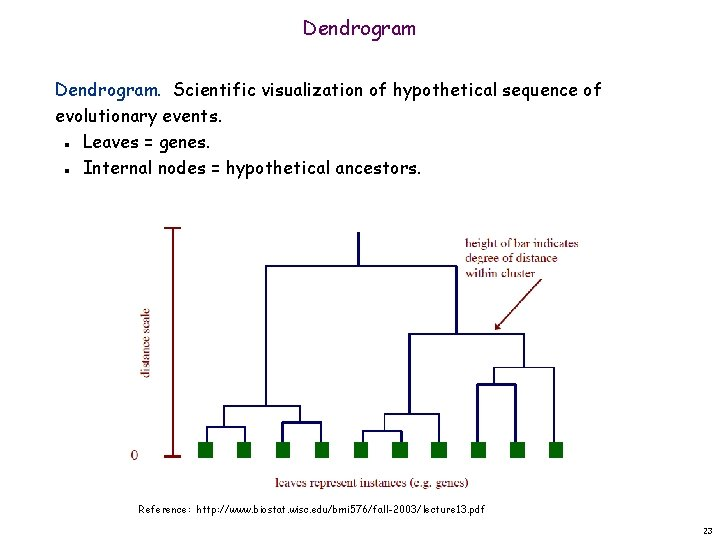Dendrogram. Scientific visualization of hypothetical sequence of evolutionary events. Leaves = genes. Internal nodes = hypothetical ancestors. n n Reference: http: //www. biostat. wisc. edu/bmi 576/fall-2003/lecture 13. pdf 23Dendrogram of Cancers in Human Tumors in similar tissues cluster together. Gene 1 Gene n Reference: Botstein & Brown group gene expressed gene not expressed 24